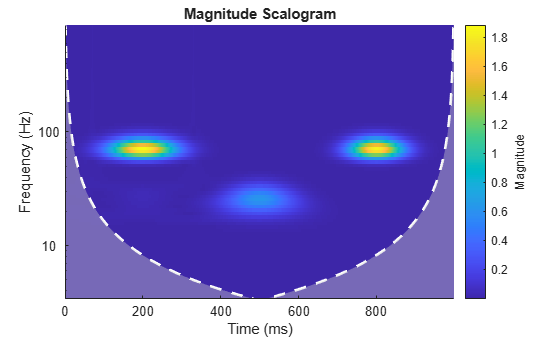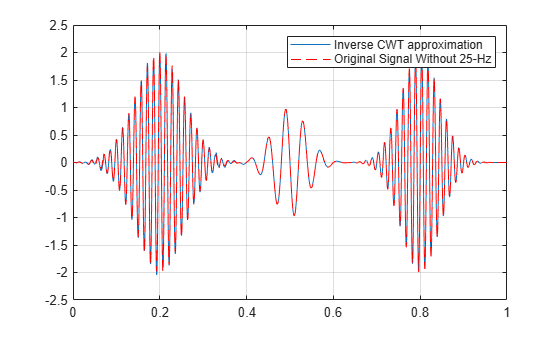# Remove Time-Localized Frequency Components

Create a signal consisting of exponentially weighted sine waves. The signal has two 25-Hz components -- one centered at 0.2 seconds and one centered at 0.5 seconds. It also has two 70-Hz components -- one centered at 0.2 and one centered at 0.8 seconds. The first 25-Hz and 70-Hz components co-occur in time.

```t = 0:1/2000:1-1/2000; dt = 1/2000; x1 = sin(50*pi*t).*exp(-50*pi*(t-0.2).^2); x2 = sin(50*pi*t).*exp(-100*pi*(t-0.5).^2); x3 = 2*cos(140*pi*t).*exp(-50*pi*(t-0.2).^2); x4 = 2*sin(140*pi*t).*exp(-80*pi*(t-0.8).^2); x = x1+x2+x3+x4; plot(t,x) grid on; title('Superimposed Signal')```Obtain and display the CWT.

```cwt(x,2000); title('Analytic CWT using Default Morse Wavelet');```Remove the 25 Hz component which occurs from approximately 0.07 to 0.3 seconds by zeroing out the CWT coefficients. Use the inverse CWT (`icwt`) to reconstruct an approximation to the signal.

```[cfs,f] = cwt(x,2000); T1 = .07; T2 = .33; F1 = 19; F2 = 34; cfs(f > F1 & f < F2, t> T1 & t < T2) = 0; xrec = icwt(cfs);```

Display the CWT of the reconstructed signal. The initial 25-Hz component is removed.

`cwt(xrec,2000)`Plot the original signal and the reconstruction.

```subplot(2,1,1); plot(t,x); grid on; title('Original Signal'); subplot(2,1,2); plot(t,xrec) grid on; title('Signal with first 25-Hz component removed');```Compare the reconstructed signal with the original signal without the 25-Hz component centered at 0.2 seconds.

```y = x2+x3+x4; figure; plot(t,xrec) hold on plot(t,y,'r--') grid on; legend('Inverse CWT approximation','Original Signal Without 25-Hz'); hold off```